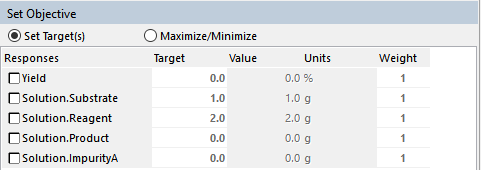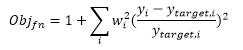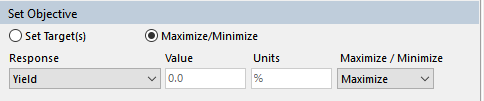Scale-up Suite Help
Set objective function

There are two main ways of optimizing in DynoChem. These are:

1. Using Set targets. This allows you to set a target value for either a single or multiple responses (e.g. find conditions where the yield is 90% and the substrate residual is 0.5%). This effectively builds an objective function for you. This allows multiple objectives to be achieved without the need to write complex objective functions in calculate statements on the process sheet.
2. Using Maximize/Minimize. This allows you to find the maximum or minimum value of a single response (e.g. Yield). Complex objective functions (written as calculate statements on the process sheet of the model) can be optimized using this approach.
Using Set TargetsThe variables that appear in the Responses list are as follows:

• Variables called ‘Obj_fn’, ’Yield’ or ‘Selectivity’ (these will be automatically chosen in this order of priority as the objective function). These variables will have been set up using Calculate statements on the Process sheet of the Excel model
• All variables that appeared on the left hand side of a Calculate statement in the Excel model process sheet
• All species variables in each phase.
Select one or more Responses that you want to achieve a target. For example this may be a yield of 90% and a substrate residual of 0.5%. Type the target values in the column marked "Target".

For targets, the objective function is based on the square of the distance from the target value. The function to be minimized is:This value is plotted in the chart as a solid black line when optimizing.

If there is more than one Target, weight the parameters according to their relative importance. A high weighting (larger than 1) will mean that the optimization routine will work harder to meet that target than other targets with lower weighting.

Using Maximize/Minimize

When you select this option in Optimization, the list of potential responses (the variables that can be optimized) appear in a dropdown menu of the Select Objective function interface.The variables that appear in the response dropdown list are as follows:

• Variables called 'Obj_fn', 'Yield' or 'Selectivity' (these will be automatically chosen in this order of priority as the objective function). These variables will have been set up using Calculate statements on the Process sheet of the Excel model.
• All variables that appeared on the left hand side of a Calculate statement in the Excel model process sheet.
• All species variables in each phase.

To define your goal for an optimization exercise:

1. Select an objective using the Response dropdown
2. Specify whether the objective is to be maximized or minimized using the Maximize/ Minimize dropdown.

Note: the Value cell will initially be empty on setting up an Optimization exercise, but is updated with the latest value of the objective function as the model runs. The Units of the objective function are set on the process sheet of the model.